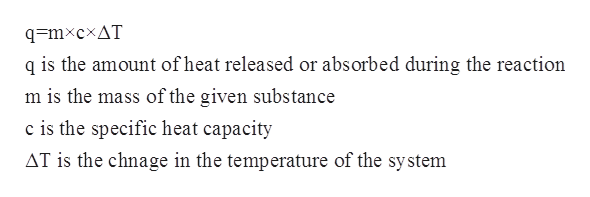# It takes 45.0 J to raise the temperature of an 11.8 g piece of unknown metal from 13.0∘C to 24.5 ∘C. What is the specific heat for the metal?

Question
46 views

It takes 45.0 J to raise the temperature of an 11.8 g piece of unknown metal from 13.0∘C to 24.5 ∘C. What is the specific heat for the metal?

check_circle

Step 1

The specific heat capacity for a given substance refers to the amount of heat that is needed to raise the temperature of a given substance per unit of its mass. The mathematical relationship between specific heat capacity, mass, change in temperature and the heat of the solution is given by the following equation:help_outlineImage Transcriptioncloseq=mxcxAT q is the amount of heat released or absorbed during the reaction m is the mass of the given substance c is the specific heat capacity AT is the chnage in the temperature of the system fullscreen
Step 2

In this problem, it has been given that the mass of the unknown metal is 11.8 g.

The change in temperature is given to be from 13oC to 24.5oC which can be calculated as follows:

Step 3

The heat of the temperature is given to be 45.0 J

Substitutin...

### Want to see the full answer?

See Solution

#### Want to see this answer and more?

Solutions are written by subject experts who are available 24/7. Questions are typically answered within 1 hour.*

See Solution
*Response times may vary by subject and question.
Tagged in

### Chemistry